#### Topological considerations

So far there has been no explicit consideration of the topology of. Assuming thatis a manifold, the concepts discussed so far can be applied to any open set on which coordinates are defined. In practice, it is often convenient to use the manifold representations of Section 4.1.2. The manifold can be expressed as a cube,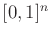, with some faces identified to obtain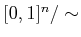. Over the interior of the cube, all of the concepts explained in this section work without modification. At the boundary, the samples used for interpolation must take the identification into account. Furthermore, actions,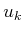, and next states,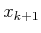, must function correctly on the boundary. One must be careful, however, in declaring that some solution is optimal, because Euclidean shortest paths depend on the manifold parameterization. This ambiguity is usually resolved by formulating the cost in terms of some physical quantity, such as time or energy. This often requires modeling dynamics, which will be covered in Part IV.

Value iteration with interpolation is extremely general. It is a generic algorithm for approximating the solution to optimal control problems. It can be applied to solve many of the problems in Part IV by restricting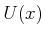to take into account complicated differential constraints. The method can also be extended to problems that involve explicit uncertainty in predictability. This version of value iteration is covered in Section 10.6.

Steven M LaValle 2020-08-14